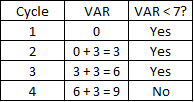## VAR Code

Consider a segment of a computer pseudocode below. What is the value of VAR at the conclusion of the script?
VAR=0
IF VAR < 7 THEN VAR = VAR + 3
ELSE EXIT LOOP
LOOP

Hint
The code is running a loop. VAR increases incrementally every time the code cycles through.
Hint 2
Factor in the exit criteria for the loop to end. The variable assigned to VAR that causes the loop to end is the answer.
The code is running a loop. VAR increases incrementally every time the code cycles through. Running through the script logically:Loop is exited once VAR = 9
VAR = 9